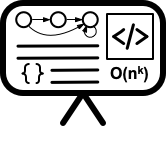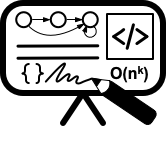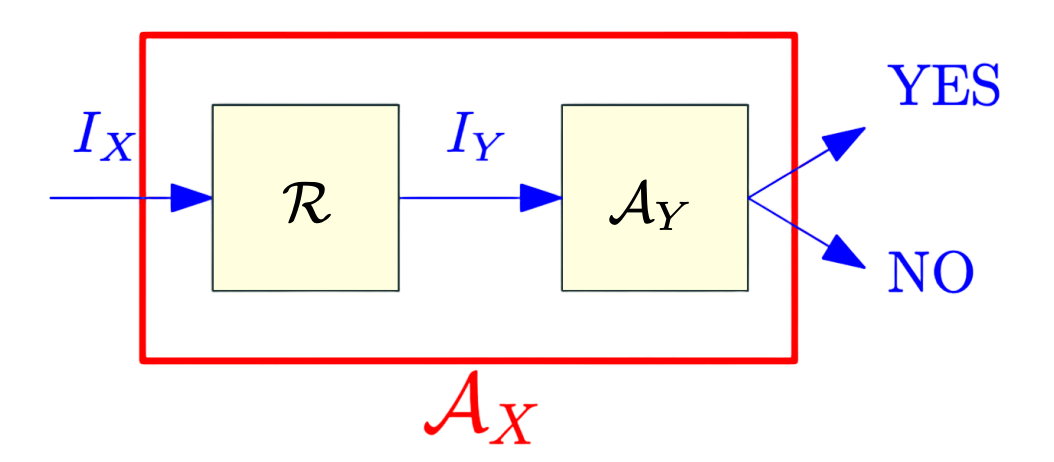# Lecture 19 - Reductions

Date Pre-lecture slides Post-lecture scribbles Lecture recording
November 02 2023## Notes

##### Introduction

In algorithms we reduce a new problem to known solved one!

###### Reductions for decision problems | languages
• R : Reduction X → Y
• $A_Y$ : Algorithm for Y
• $A_X$: New algorithm for X
• $I_X$: Instance of X
• $I_Y$: Instance of YWe can say that if R and $A_Y$ are polynomial-time algorithms, $A_X$ is also polynomial-time.

• Reductions allow us to formalize the notion of “Problem X is no harder to solve than Problem Y”
• If Problem X reduces to Problem Y (we write X $\leq$ Y), then X cannot be harder to solve than Y
• More generally, if X $\leq$ Y, we can say that X is no harder than Y, or Y is at least as hard as X. X $\leq$ Y:
• X is no harder than Y, or
• Y is at least as hard as X
##### Examples of Reduction
###### Independent Sets and Cliques

Given a graph G, a set of vertices V’ is:

• An Independent set: if no two vertices of V’ are connected by an edge of G.
• Clique : if every pair of vertices in V’ is connected by an edge of G.

We can reduce Independent Set to Clique. An instance of Independent Set is a graph G and an integer k. The reduction given $\langle G, k\rangle$ outputs $\langle G’, k\rangle$ where G’ is the complement of G. G’ has an edge uv ↔ uv is not an edge of G. So,

G has an independent set of size k ↔ G’ has a clique of size k.

• Independent Set $\leq$$_P Clique. • Clique is at least as hard as Independent Set. To show Clique \leq$$_P$ Independent Set :

Reduction figure:• $I_X$ = $\langle G’ \rangle$
• $A_X$ = Clique
• $I_Y$ = $\langle G\rangle$
• $A_Y$ = Independent Set
• R : G’ = {V, E’}

Clique and Independent Set are polynomial-time equivalent because:

• Independent Set $\leq$$_P Clique • Clique \leq$$_P$ Independent Set
###### Independent Set and Vertex Cover

Given a graph G = (V, E), a set of vertices S is:

• A vertex cover if every e $\in$ E has at least one endpoint in S

Let G = (V, E) be a graph. S is an Independent Set ↔ V \ S is a vertex cover.

To show Independent Set $\leq$$_P Vertex Cover: • G : graph with n vertices, and an integer k be an instance of the Independent Set problem. • G has an independent set of size \geq k ↔ G has a vertex cover of size \leq n-k Reduction figure:• I_X = \langle G\rangle • A_X = Independent Set(G, k) • I_Y = \langle G\rangle • A_Y = Vertex Cover(G, n-k) • R : G’ = G (G, k) is an instance of Independent Set, and (G, n - k) is an instance of Vertex Cover with the same answer.Therefore, • Independent Set \leq$$_P$ Vertex Cover
• Vertex Cover $\leq$$_P Independent Set ##### NFAs | DFAs and Universality A DFA M is universal if it accepts every string. That is, L(M) = \Sigma^*, the set of all strings. • To solve DFA Universality, we check if a DFA M has any reachable non-final state. A NFA N is said to be universal if it accepts every string. That is, L(N) = \Sigma^*, the set of all strings. • To we solve NFA Universality, we reduce it to DFA Universality. • Given an NFA N, convert it to an equivalent DFA M, and use the DFA Universality Algorithm. • The above reduction takes exponential time. • NFA Universality is known to be PSPACE-Complete. ##### Polynomial-time reductions We say that an algorithm is efficient if it runs in polynomial-time. A polynomial time reduction from a decision problem X to a decision problem Y is an algorithm A that has the following properties: • Given an instance I_X of X, A produces an instance I_Y of Y • A runs in time polynomial in |I_X|. • Answer to I_X YES ↔ answer to I_Y is YES. If X \leq$$_P$ Y then a polynomial time algorithm for Y implies a polynomial time algorithm for X.

Such a reduction is called a Karp reduction. Karp reductions are the same as mapping reductions when specialized to polynomial time for the reduction step.

• Textbooks
• Erickson, Jeff. Algorithms
• Sipser, Michael. Introduction to the Theory of Computation
• Chapter 7 - Time Complexity
• Skiena, Steven. The Algorithms Design Manual
• Chapter 11 - NP-completeness
• Sedgewick, Robert and Wayne, Kevin. Algorithms (Forth Edition)
• Chapter 6 - Context.Reductions
• Cormen, Thomas, et al. Algorithms (Forth Edition)
• Chapter 32 - NP-Completeness
• Sariel’s Lecture 21